# Decimals - Ordering Decimal Numbers

### Children normally have no problems with ordering whole numbers, but as soon as decimal fractions are included, difficulties arise.

To order decimals, we look at the largest value first, which is the leftmost column and then move column by column to the right. Comparing the the place value of the digits.

Problems arise when we move to the right of the decimal point - take the numbers 0.35 and 0.305 - children often read these as "zero point thirty five" and "zero point three hundred and five" - "three hundred and five" sounds larger than "thirty five" so they think that 0.305 is larger than .35. This is why it is important to read decimal fractions correctly - 0.35 is "zero point three five" and 0.305 is "zero point three zero five".

A typical question could be: Put these numbers in order, smallest first,
2.5, 2.05, 1.52, 0.25, 0.205, 25.25, 20.5, 1.25, 0.152

A good idea is to look at the whole number part first and group them together in order.

0.25, 0.205, 0.152      1.52, 1.25     2.5, 2.05     20.5     25.25

We now have the numbers in whole number order and need to look at the
decimal fraction part.

Take each group of whole numbers and write them below each other, making sure to line up the decimal points. Add zero's to keep the number lengths equal.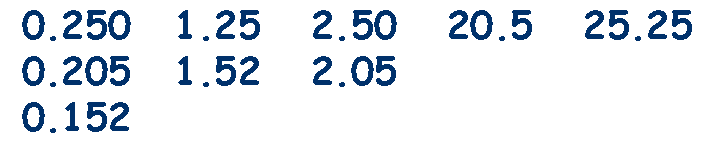Work through these groups from left to right, comparing the place value columns.

In the first group look at the tenths column first, one number is lower than the others, 0.152, score it out and write it down below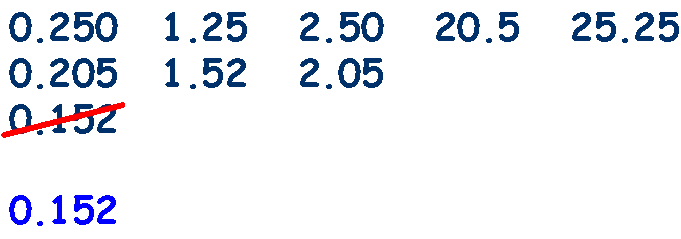We now have two numbers left in that group, both with 2 in the 1/10's column - look at the 1/1oo's column, which number has the lowest value, 0.205, score it out and write it down below. This only leaves 0.25 in that group so score it out and write it down below.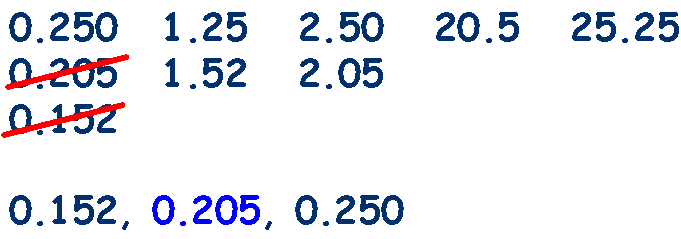Move on to the next group and repeat the procedure,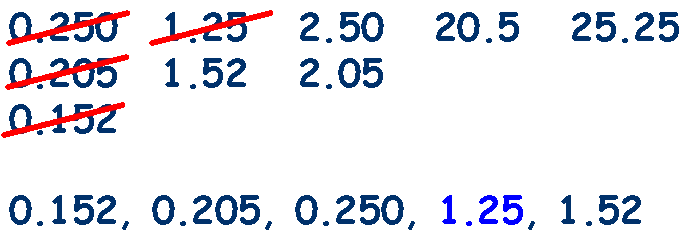Move on to the next group and repeat the procedure,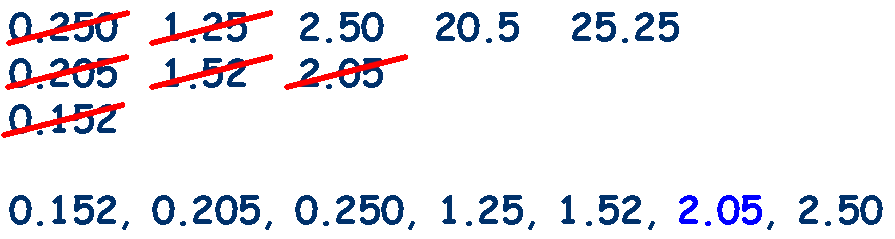Repeat this procedure until all the groups have been ordered, adding to the list as you go.## 0.152, 0.205, 0.250, 1.25, 1.52, 2.05, 2.50, 20.5, 25.25

Practice with these worksheets from Mathsblog

Ordering Decimal Fractions from Primary Resources

Burst the balloons in the correct order with this
Ordering Decimals game
.